Скачать презентацию Microwave polarization plasma diagnostic Head of group Prof

b05839911018b8d796b2d68702cf417c.ppt

• Количество слайдов: 26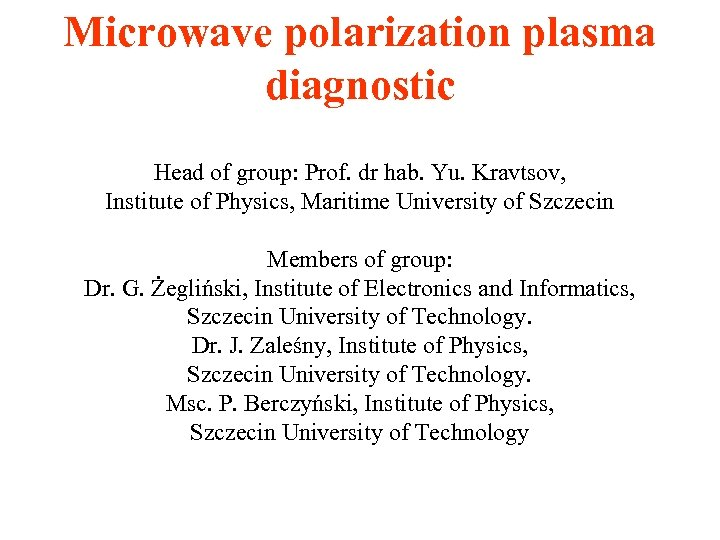Microwave polarization plasma diagnostic Head of group: Prof. dr hab. Yu. Kravtsov, Institute of Physics, Maritime University of Szczecin Members of group: Dr. G. Żegliński, Institute of Electronics and Informatics, Szczecin University of Technology. Dr. J. Zaleśny, Institute of Physics, Szczecin University of Technology. Msc. P. Berczyński, Institute of Physics, Szczecin University of Technology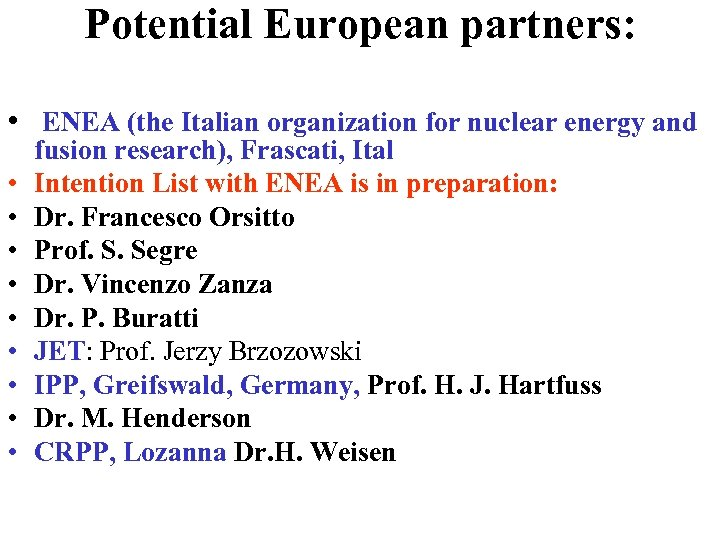Potential European partners: • ENEA (the Italian organization for nuclear energy and • • • fusion research), Frascati, Ital Intention List with ENEA is in preparation: Dr. Francesco Orsitto Prof. S. Segre Dr. Vincenzo Zanza Dr. P. Buratti JET: Prof. Jerzy Brzozowski IPP, Greifswald, Germany, Prof. H. J. Hartfuss Dr. M. Henderson CRPP, Lozanna Dr. H. Weisen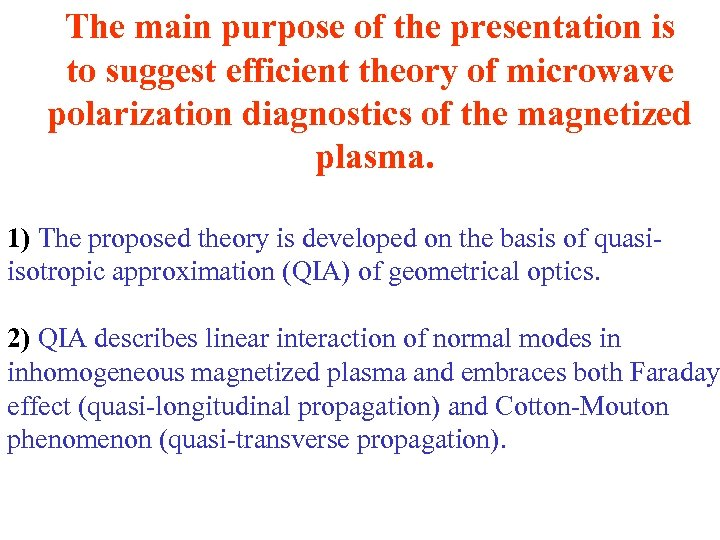The main purpose of the presentation is to suggest efficient theory of microwave polarization diagnostics of the magnetized plasma. 1) The proposed theory is developed on the basis of quasiisotropic approximation (QIA) of geometrical optics. 2) QIA describes linear interaction of normal modes in inhomogeneous magnetized plasma and embraces both Faraday effect (quasi-longitudinal propagation) and Cotton-Mouton phenomenon (quasi-transverse propagation).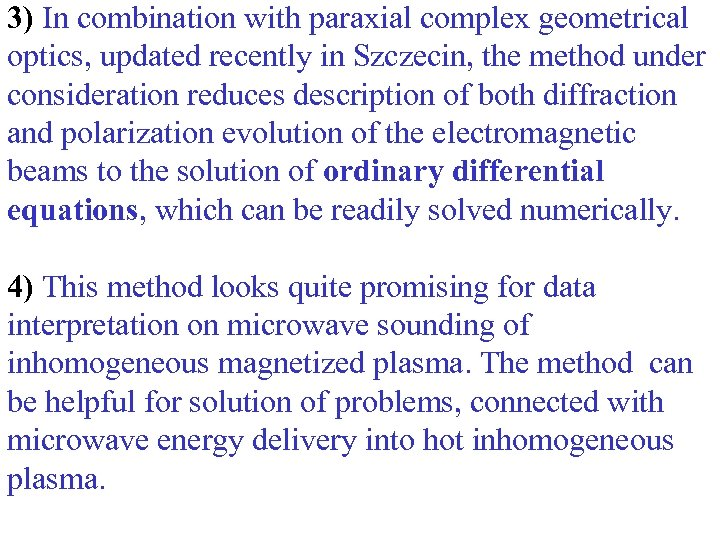3) In combination with paraxial complex geometrical optics, updated recently in Szczecin, the method under consideration reduces description of both diffraction and polarization evolution of the electromagnetic beams to the solution of ordinary differential equations, which can be readily solved numerically. 4) This method looks quite promising for data interpretation on microwave sounding of inhomogeneous magnetized plasma. The method can be helpful for solution of problems, connected with microwave energy delivery into hot inhomogeneous plasma.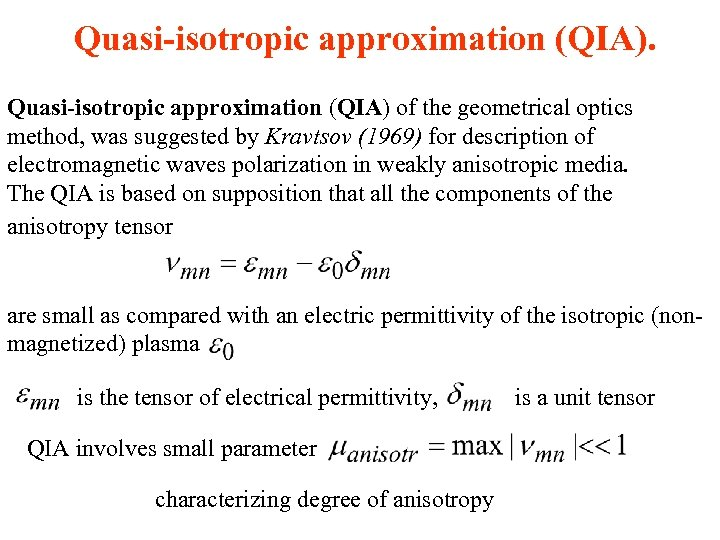Quasi-isotropic approximation (QIA) of the geometrical optics method, was suggested by Kravtsov (1969) for description of electromagnetic waves polarization in weakly anisotropic media. The QIA is based on supposition that all the components of the anisotropy tensor are small as compared with an electric permittivity of the isotropic (nonmagnetized) plasma is the tensor of electrical permittivity, QIA involves small parameter characterizing degree of anisotropy is a unit tensor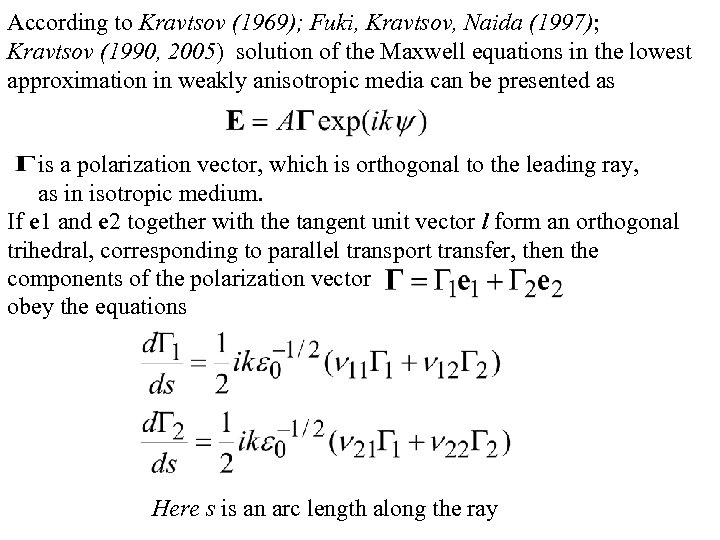According to Kravtsov (1969); Fuki, Kravtsov, Naida (1997); Kravtsov (1990, 2005) solution of the Maxwell equations in the lowest approximation in weakly anisotropic media can be presented as is a polarization vector, which is orthogonal to the leading ray, as in isotropic medium. If e 1 and e 2 together with the tangent unit vector l form an orthogonal trihedral, corresponding to parallel transport transfer, then the components of the polarization vector obey the equations Here s is an arc length along the ray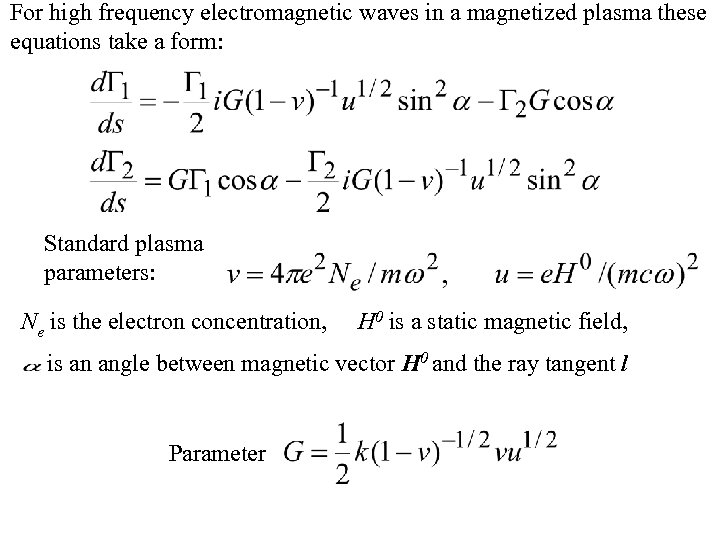For high frequency electromagnetic waves in a magnetized plasma these equations take a form: Standard plasma parameters: Ne is the electron concentration, H 0 is a static magnetic field, is an angle between magnetic vector H 0 and the ray tangent l Parameter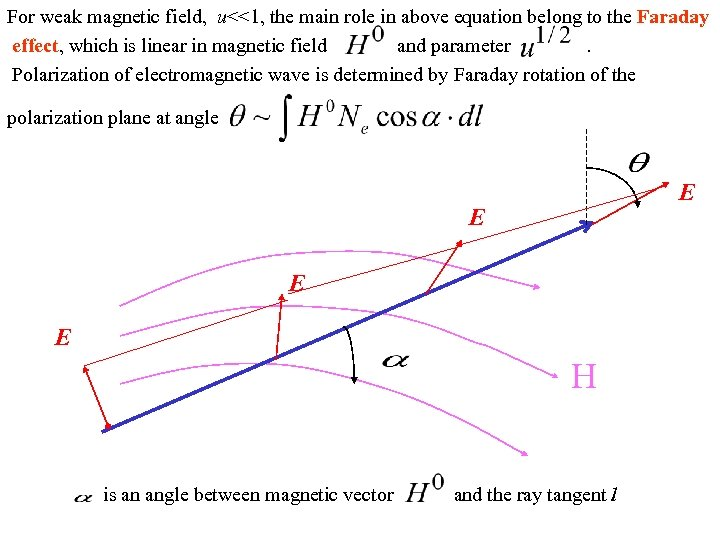For weak magnetic field, u<<1, the main role in above equation belong to the Faraday effect, which is linear in magnetic field and parameter. Polarization of electromagnetic wave is determined by Faraday rotation of the polarization plane at angle E E H is an angle between magnetic vector and the ray tangent l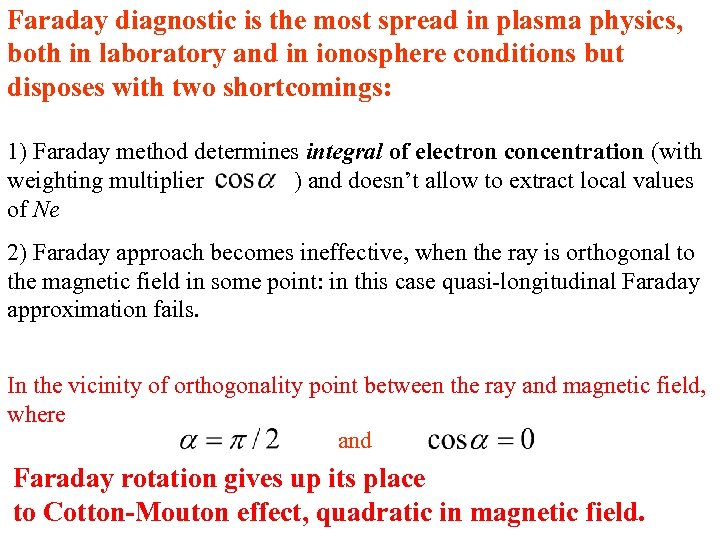Faraday diagnostic is the most spread in plasma physics, both in laboratory and in ionosphere conditions but disposes with two shortcomings: 1) Faraday method determines integral of electron concentration (with weighting multiplier ) and doesn’t allow to extract local values of Ne 2) Faraday approach becomes ineffective, when the ray is orthogonal to the magnetic field in some point: in this case quasi-longitudinal Faraday approximation fails. In the vicinity of orthogonality point between the ray and magnetic field, where and Faraday rotation gives up its place to Cotton-Mouton effect, quadratic in magnetic field.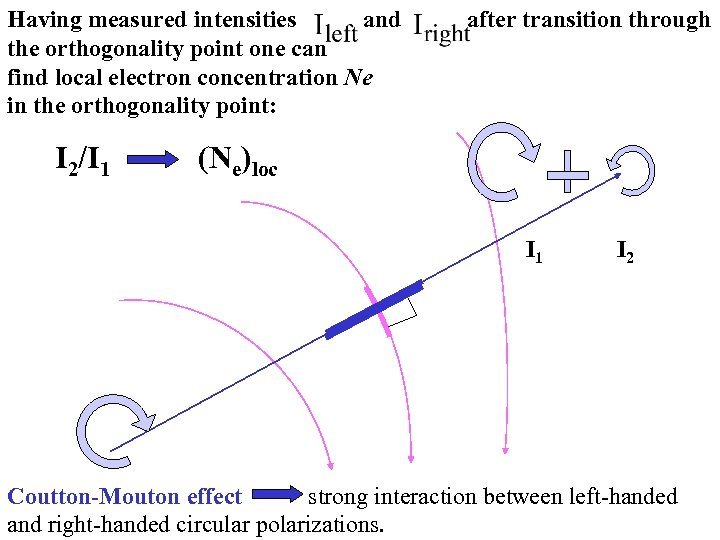Having measured intensities and after transition through the orthogonality point one can find local electron concentration Ne in the orthogonality point: I 2/I 1 (Ne)loc I 1 I 2 Coutton-Mouton effect strong interaction between left-handed and right-handed circular polarizations.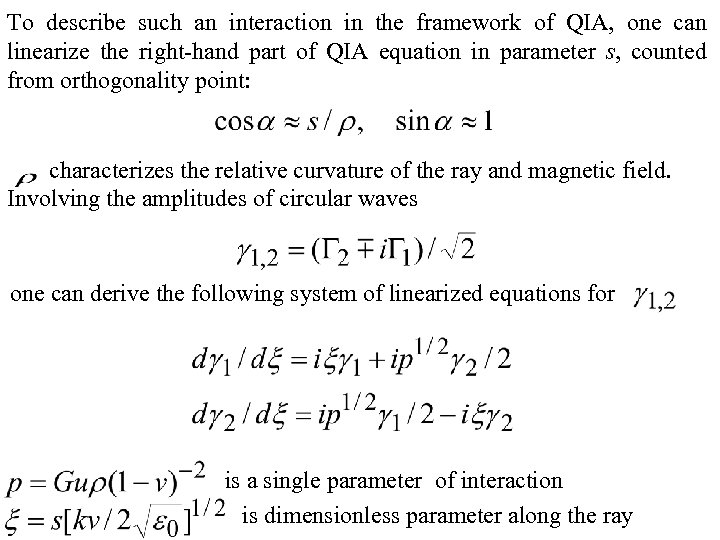To describe such an interaction in the framework of QIA, one can linearize the right-hand part of QIA equation in parameter s, counted from orthogonality point: characterizes the relative curvature of the ray and magnetic field. Involving the amplitudes of circular waves one can derive the following system of linearized equations for is a single parameter of interaction is dimensionless parameter along the ray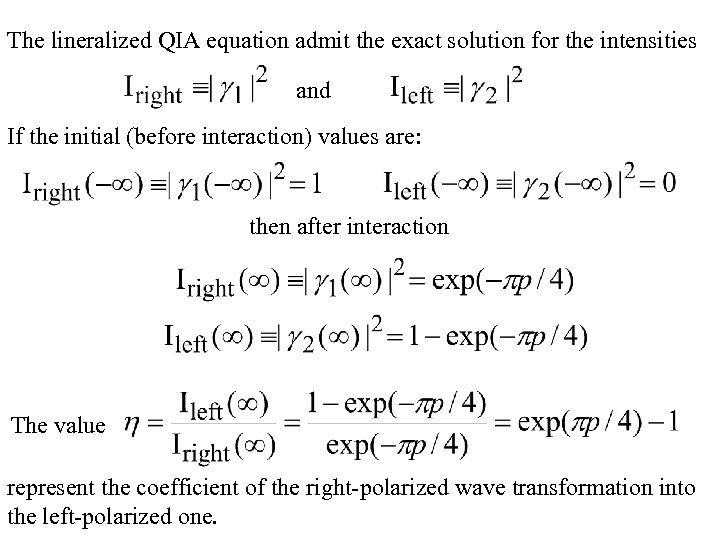The lineralized QIA equation admit the exact solution for the intensities and If the initial (before interaction) values are: then after interaction The value represent the coefficient of the right-polarized wave transformation into the left-polarized one.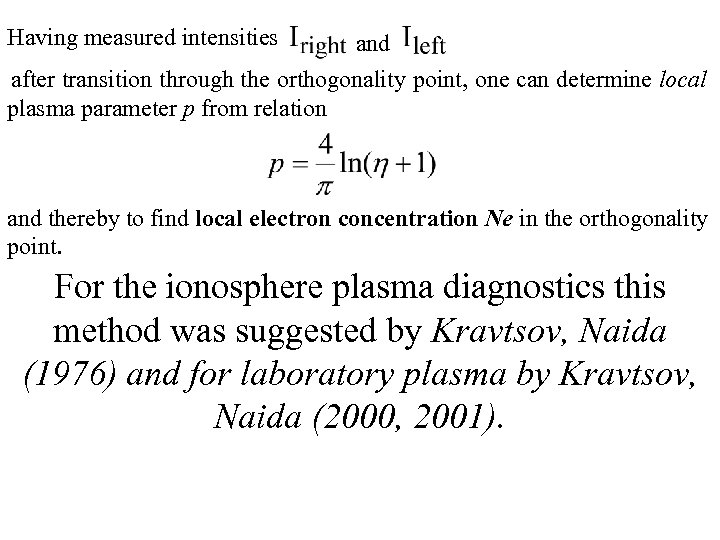Having measured intensities and after transition through the orthogonality point, one can determine local plasma parameter p from relation and thereby to find local electron concentration Ne in the orthogonality point. For the ionosphere plasma diagnostics this method was suggested by Kravtsov, Naida (1976) and for laboratory plasma by Kravtsov, Naida (2000, 2001).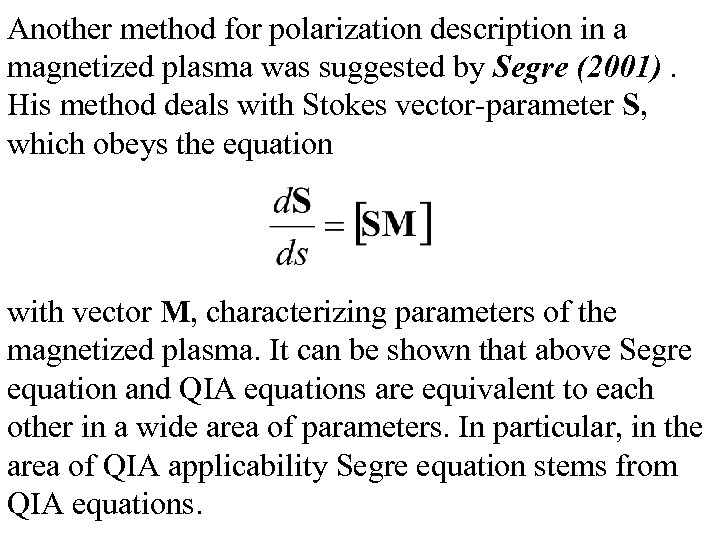Another method for polarization description in a magnetized plasma was suggested by Segre (2001). His method deals with Stokes vector-parameter S, which obeys the equation with vector M, characterizing parameters of the magnetized plasma. It can be shown that above Segre equation and QIA equations are equivalent to each other in a wide area of parameters. In particular, in the area of QIA applicability Segre equation stems from QIA equations.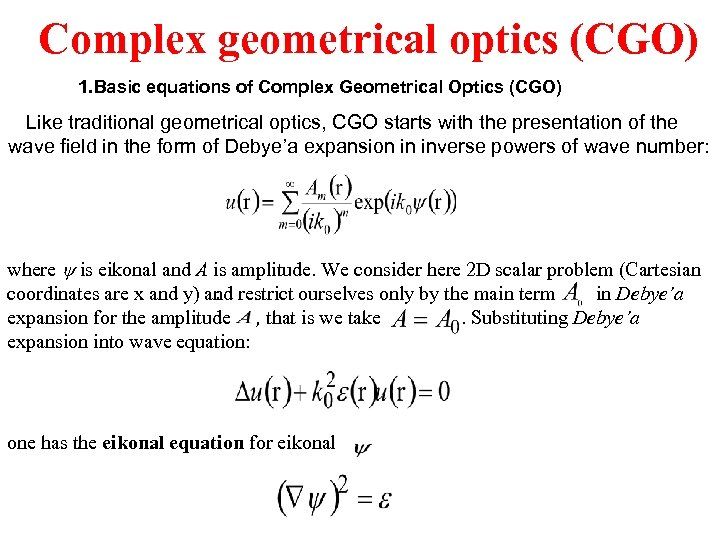Complex geometrical optics (CGO) 1. Basic equations of Complex Geometrical Optics (CGO) Like traditional geometrical optics, CGO starts with the presentation of the wave field in the form of Debye’a expansion in inverse powers of wave number: where is eikonal and A is amplitude. We consider here 2 D scalar problem (Cartesian : coordinates are x and y) and restrict ourselves only by the main term in Debye’a expansion for the amplitude , that is we take. Substituting Debye’a expansion into wave equation: one has the eikonal equation for eikonal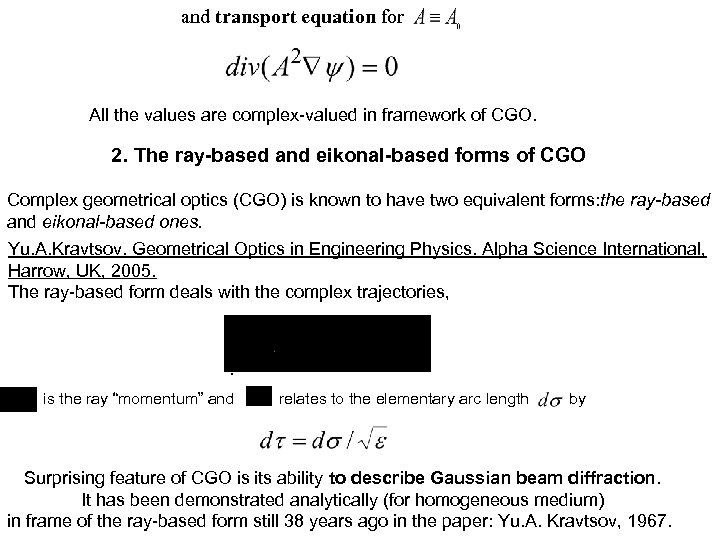and transport equation for All the values are complex-valued in framework of CGO. 2. The ray-based and eikonal-based forms of CGO Complex geometrical optics (CGO) is known to have two equivalent forms: the ray-based and eikonal-based ones. Yu. A. Kravtsov. Geometrical Optics in Engineering Physics. Alpha Science International, Harrow, UK, 2005. The ray-based form deals with the complex trajectories, . is the ray “momentum” and relates to the elementary arc length by Surprising feature of CGO is its ability to describe Gaussian beam diffraction. It has been demonstrated analytically (for homogeneous medium) in frame of the ray-based form still 38 years ago in the paper: Yu. A. Kravtsov, 1967.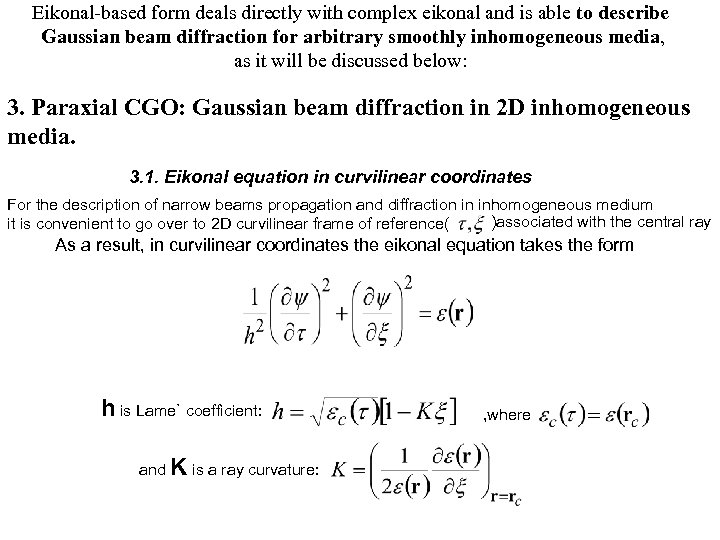Eikonal-based form deals directly with complex eikonal and is able to describe Gaussian beam diffraction for arbitrary smoothly inhomogeneous media, as it will be discussed below: 3. Paraxial CGO: Gaussian beam diffraction in 2 D inhomogeneous media. 3. 1. Eikonal equation in curvilinear coordinates For the description of narrow beams propagation and diffraction in inhomogeneous medium )associated with the central ray it is convenient to go over to 2 D curvilinear frame of reference( As a result, in curvilinear coordinates the eikonal equation takes the form h is Lame` coefficient: and K is a ray curvature: , where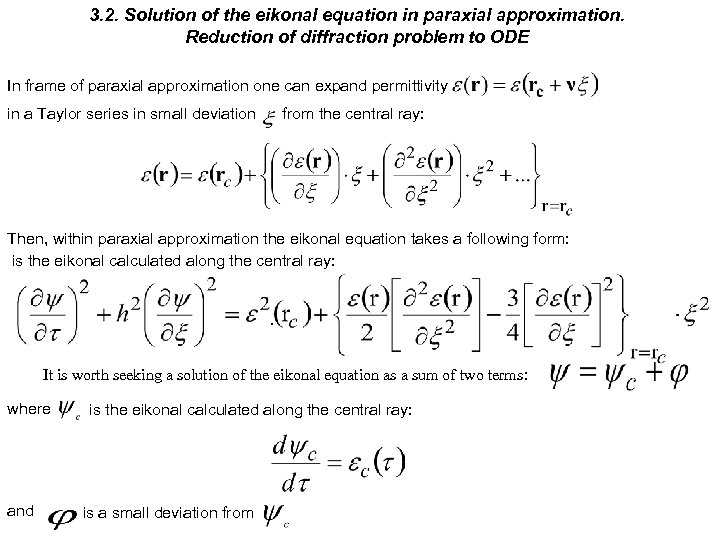3. 2. Solution of the eikonal equation in paraxial approximation. Reduction of diffraction problem to ODE In frame of paraxial approximation one can expand permittivity in a Taylor series in small deviation from the central ray: Then, within paraxial approximation the eikonal equation takes a following form: is the eikonal calculated along the central ray: . It is worth seeking a solution of the eikonal equation as a sum of two terms: where and is the eikonal calculated along the central ray: is a small deviation from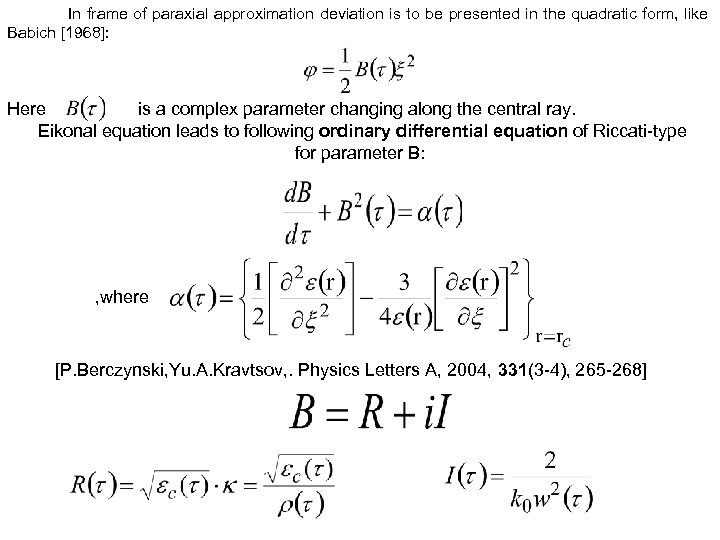In frame of paraxial approximation deviation is to be presented in the quadratic form, like Babich : Here is a complex parameter changing along the central ray. Eikonal equation leads to following ordinary differential equation of Riccati-type for parameter B: , where [P. Berczynski, Yu. A. Kravtsov, . Physics Letters A, 2004, 331(3 -4), 265 -268]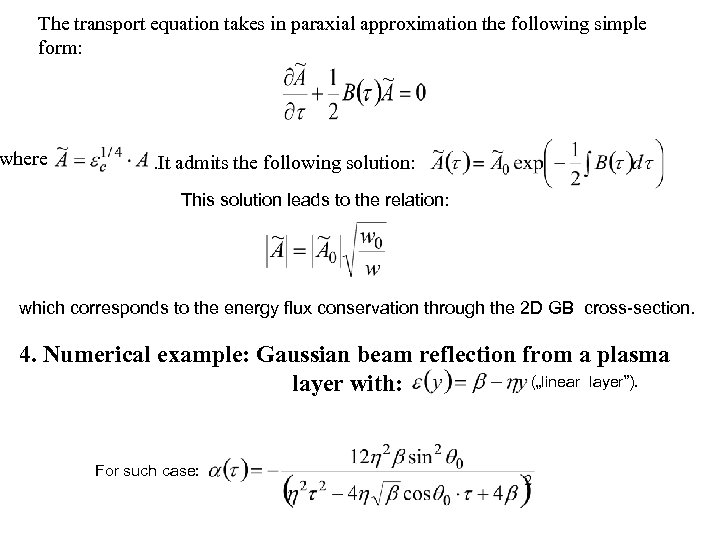The transport equation takes in paraxial approximation the following simple form: where . It admits the following solution: This solution leads to the relation: which corresponds to the energy flux conservation through the 2 D GB cross-section. 4. Numerical example: Gaussian beam reflection from a plasma („linear layer”). layer with: For such case: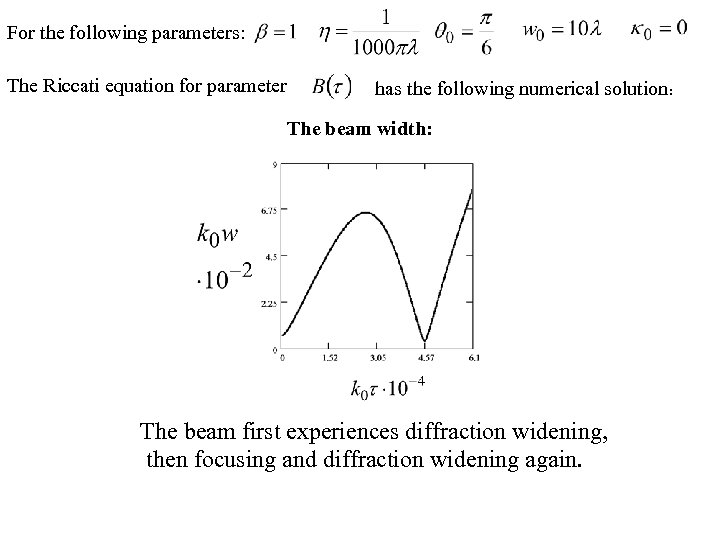For the following parameters: The Riccati equation for parameter has the following numerical solution: The beam width: The beam first experiences diffraction widening, then focusing and diffraction widening again.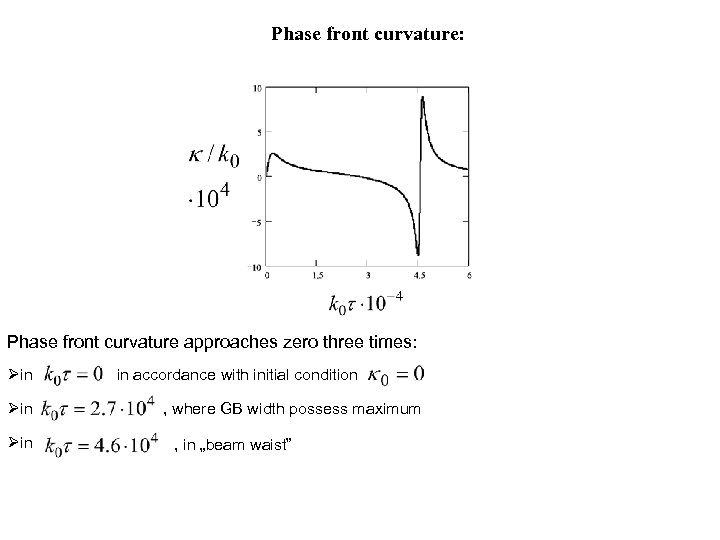Phase front curvature: Phase front curvature approaches zero three times: in in accordance with initial condition , where GB width possess maximum , in „beam waist”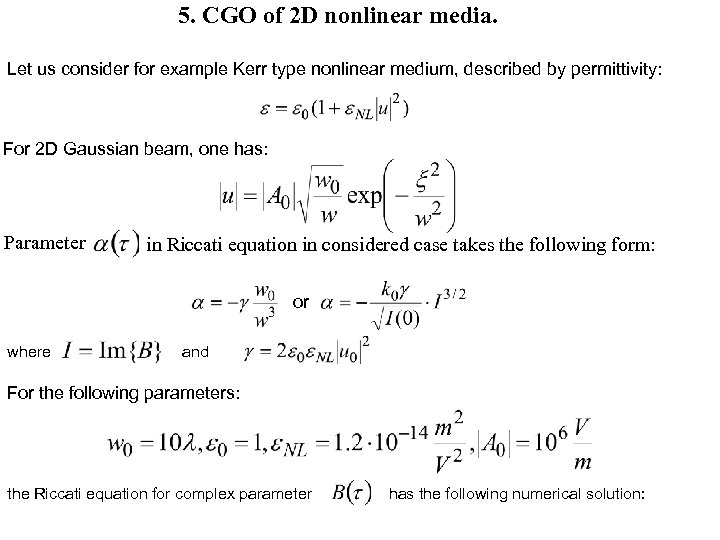5. CGO of 2 D nonlinear media. Let us consider for example Kerr type nonlinear medium, described by permittivity: For 2 D Gaussian beam, one has: Parameter in Riccati equation in considered case takes the following form: or where and For the following parameters: the Riccati equation for complex parameter has the following numerical solution: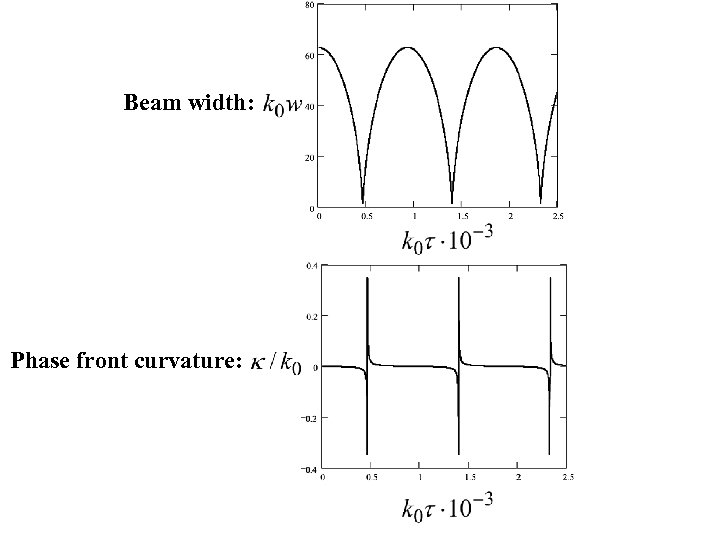Beam width: Phase front curvature: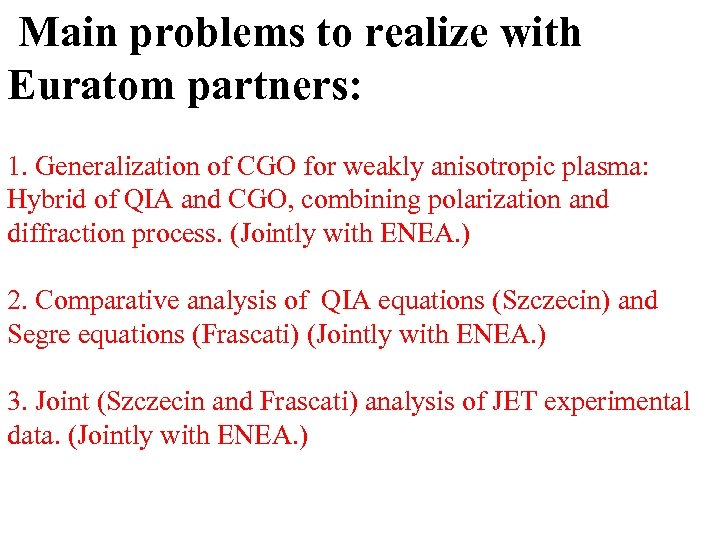Main problems to realize with Euratom partners: 1. Generalization of CGO for weakly anisotropic plasma: Hybrid of QIA and CGO, combining polarization and diffraction process. (Jointly with ENEA. ) 2. Comparative analysis of QIA equations (Szczecin) and Segre equations (Frascati) (Jointly with ENEA. ) 3. Joint (Szczecin and Frascati) analysis of JET experimental data. (Jointly with ENEA. )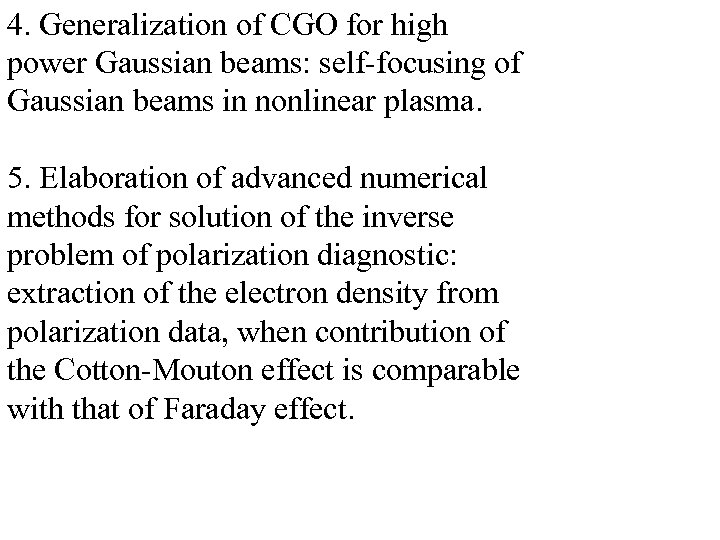4. Generalization of CGO for high power Gaussian beams: self-focusing of Gaussian beams in nonlinear plasma. 5. Elaboration of advanced numerical methods for solution of the inverse problem of polarization diagnostic: extraction of the electron density from polarization data, when contribution of the Cotton-Mouton effect is comparable with that of Faraday effect.